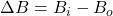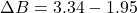## The magnetic coils of a tokamak fusion reactor are in the shape of a toroid having an inner radius of 0.700 m and an outer radius of 1.20 m.

Question

The magnetic coils of a tokamak fusion reactor are in the shape of a toroid having an inner radius of 0.700 m and an outer radius of 1.20 m. The toroid has 900 turns of large diameter wire, each of which carries a current of 13.0 kA. Find the difference in magnitudes of the magnetic fields of the toroid along the inner and outer radii. (Enter your answer in T.)

in progress 0
3 weeks 2021-08-22T18:32:39+00:00 1 Answers 0 views 0

The difference is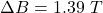Explanation:

From the question we are told that

The inner radius  is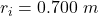The outer radius  is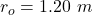The number of turns is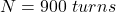The current on each wire is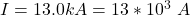Generally magnetic field of a toroid along the outer radius is mathematically evaluated as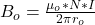Where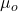is the permeability of free space with value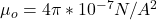substituting values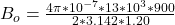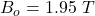Generally magnetic field of a toroid along the inner radius is mathematically evaluated as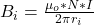substituting values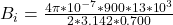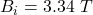The difference in  magnitudes of the magnetic fields of the toroid along the inner and outer radii is mathematically evaluated as